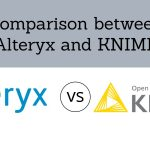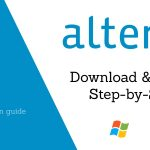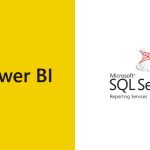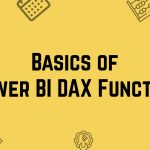[email protected] +1 203-349-9909 / +91-8080042523

Excel Formula

### How to use the Excel Choose Function

The CHOOSE Function in Excel returns a value from a list using a given position or index. The values provided to CHOOSE can include references. Let’s have a look below to get more details about CHOOSE Function. ➡ What is Excel Choose Function? CHOOSE Function is a function in Excel that helps you to get...

### How to use the Excel Code Function

The CODE Function in Excel returns a numeric code for a given character. The CODE Function can be used to understand numeric codes given to the CHAR Function as it is the reverse process of CHAR Function. ➡ What is Code Function in Excel? The Function that accepts one argument, for the text for which you...

### How to use the Excel Column Function

The COLUMN function in Excel returns the column number for a reference. In other words, Column Function too will return the column number of the cell which contains formula when reference is not provided. ➡ What is Column Function in Excel? The function which will returns column number for a reference is called Excel COLUMN Function....

### How to use Char Function in Excel

The CHAR Function in Excel returns a character when given a valid Character Code. CHAR Function is used to specify the characters that are difficult to enter in a formula in Excel. Now, let us study in detail the CHAR Function as given below. What is a CHAR Function in Excel? What is the formula...

### How to Use SUBSTITUTE Function

Let’s first grasp what a Substitute Function is and when to use it. Before we figure out how to use the SUBSTITUTE function. What is a Substitute function? A Substitute is a person or a thing acting or serving in place of another. Substitute function replaces/swaps a set of characters with another in a text string. For...

### Working with Excel Sumif Formula

When I ask professionals in my workshops – “Which is the ONE formula you’ll pick if you were asked to choose JUST ONE?” Most of the answers hover around INDEX, VLOOKUP and IF. But they tend to forget Excel Sumif. You know what MY answer is – Excel SUMIFS()! And I will tell you why. Excel Sumif...

### 9 Excel Error in Formula and How to correct it

Do you feel agitated when you are engrossed in setting up formulas in your EXCEL and suddenly you see jargon of excel error like #NAME, #REF appearing in your excel spreadsheet? Here’s a list of top 9 mistakes that one makes while entering an Excel formula + tips on how to avoid them: 1. Excel Error –...

Microsoft Excel has a lot of features one of it is excel formula auditing. It has a variety of powerful tools that allow checking what is happening in your spreadsheet. Today we will discuss on Advanced Excel formula. It helps you to get the relations between formula and your cells. Which cells are using the...